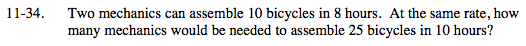Home > MC2 > Chapter 11 > Lesson 11.2.1 > Problem11-34

11-34.

Two mechanics can assemble 10 bicycles in 8 hours. At the same rate, how many mechanics would be needed to assemble 25 bicycles in 10 hours? Homework Help ✎What is the work rate of one mechanic?

Write an equation.

$\text{(rate of a mechanic)(total mechanics)}= \frac{25\text{ bicycles}}{10\text{ hours}}$

$\frac{5\text{ bicycles}}{8\text{ hours}} (x) = \frac{25\text{ bicycles}}{10\text{ hours}}$

4 mechanics